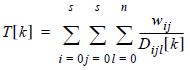# Fuzzy Methodology

## Producer Field Guide

HGD_Product
Producer Field Guide
HGD_Portfolio_Suite
Producer

Fuzzy Classification

Fuzzy Classification method takes into account that there are pixels of mixed make-up, that is, a pixel cannot be definitively assigned to one category. Jensen notes that, "Clearly, there needs to be a way to make the classification algorithms more sensitive to the imprecise (fuzzy) nature of the real world" (Jensen, 1996).

Fuzzy classification is designed to help you work with data that may not fall into exactly one category or another. Fuzzy classification works using a membership function, wherein a pixel’s value is determined by whether it is closer to one class than another. A fuzzy classification does not have definite boundaries, and each pixel can belong to several different classes (Jensen, 1996).

Like traditional classification, fuzzy classification still uses training, but the biggest difference is that "it is also possible to obtain information on the various constituent classes found in a mixed pixel. . ." (Jensen, 1996). Jensen goes on to explain that the process of collecting training sites in a fuzzy classification is not as strict as a traditional classification. In the fuzzy method, the training sites do not have to have pixels that are exactly the same.

Once you have a fuzzy classification, you can perform a moving window convolution on a fuzzy classification with multiple output class assignments using the Fuzzy Convolution dialog. Using the multilayer classification and distance file, the convolution creates a new single class output file by computing a total weighted distance for all classes in the window.

Fuzzy Convolution

Fuzzy Convolution operation creates a single classification layer by calculating the total weighted inverse distance of all the classes in a window of pixels. Then, it assigns the center pixel in the class with the largest total inverse distance summed over the entire set of fuzzy classification layers.

This has the effect of creating a context-based classification to reduce the speckle or salt and pepper in the classification. Classes with a very small distance value remain unchanged while classes with higher distance values may change to a neighboring value if there is a sufficient number of neighboring pixels with class values and small corresponding distance values. The following equation is used in the calculation:Where:

i = row index of window

j = column index of window

s = size of window (3, 5, or 7)

l = layer index of fuzzy set

n = number of fuzzy layers used

W = weight table for window

k = class value

D[k] = distance file value for class k

T[k] = total weighted distance of window for class k

The center pixel is assigned the class with the maximum T[k].